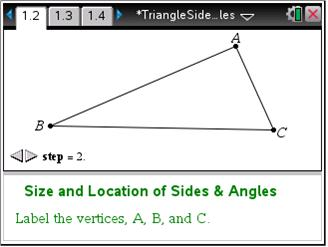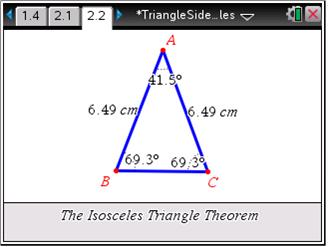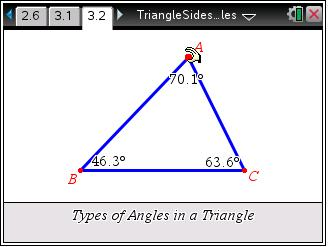# Activities

••• ##### Subject Area

• Math: Geometry: Triangles

• ##### Author9-12

40 Minutes

• ##### Device
•TI-Nspire™ CX/CX II
•TI-Nspire™ CX CAS/CX II CAS
• TI-Nspire™ Navigator™
• TI-Nspire™
• TI-Nspire™ CAS
• ##### Software

TI-Nspire™
TI-Nspire™ CAS

3.2

• ##### Other Materials
By Karen Campe

## Triangle Sides & Angles

#### Activity Overview

Students will explore side and angle relationships in a triangle. First, students will discover where the longest (and shortest) side is located relative to the largest (and smallest) angle. Then, students will explore the Isosceles Triangle Theorem and its converse. Finally, students will determine the number of acute, right, or obtuse angles that can exist in any one triangle.

#### Key Steps

•Students will construct a triangle and label the vertices A, B, and C. After measuring the angles and lengths of the sides, they will drag the vertices to make observations about the locations of the sides relative to the size of the angles.

•On page 2.2, an isosceles triangle (two sides with equal lengths) has been constructed.

Students should measure all three angles using the Angle tool, as before. They will drag a vertex around the page to make a conjecture about the angles.

•On page 3.2, triangle ABC is drawn with its angle measures displayed.

Students should drag a vertex and notice how many angles of each type can exist in a triangle.